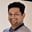Related Tags

java
binary
communitycreator

# How to convert an integer to binary in JavaTarun Telang

## Introduction

There are multiple techniques for converting an integer (i) to equivalent binary representation. This article discusses the most straightforward approach.

## Integer.toBinaryString(int i)

To convert an integer to binary, we can simply call the public static String toBinaryString(int i) method of the Integer class. This method returns the binary string that corresponds to the integer passed as an argument to this function.

The string returned from the Integer.toBinaryString(int i) method call would not have padding by 0s.

To add the padding, first format the string to fixed-width string using the String.format() method. Then, replace spaces with 0s using the String.replaceAll(String str, String replacement).

Take a look at the code snippet below:

String.format("%32s", binaryString).replaceAll(" ", "0");


For increased readability, we can add 4-bit blocks using the following code snippet.

StringBuffer sb = new StringBuffer();
for (int i = 0; i< binaryString.length(); i++ ) {
sb.append(binaryString.charAt(i));
if (i%4 == 3 && i != binaryString.length() - 1)
sb.append(separator);
}


Take a look at the code below to understand this better.

Int2Binary.java
__ed_input.txt
import java.util.*;

class Int2Binary {
private static String binaryString;

private static void int2binary(int n) {
binaryString = Integer.toBinaryString(n);
}

binaryString = String.format("%32s", binaryString).replaceAll(" ", "0");
}

private static void addBlocks(String separator) {
StringBuffer sb = new StringBuffer();
for (int i = 0; i< binaryString.length(); i++ ){
sb.append(binaryString.charAt(i));
if (i%4 == 3 && i != binaryString.length() - 1)
sb.append(separator);
}
binaryString = sb.toString();
}

public static void convert2Binary(int n) {
int2binary(n);
}

public static void main(String[] args) {
int n = 10;
for (int i = -5; i <= 5; i++){
convert2Binary(i);
System.out.format("Binary representation of %2d is %s\n", i, binaryString);
}
}
}
Integer to Binary

RELATED TAGS

java
binary
communitycreator

CONTRIBUTORTarun Telang
RELATED COURSES

View all Courses

Keep Exploring

Learn in-demand tech skills in half the time